# 22. Impure nickel, refined by smelting sulfide ores in a blast furnace, can be converted into...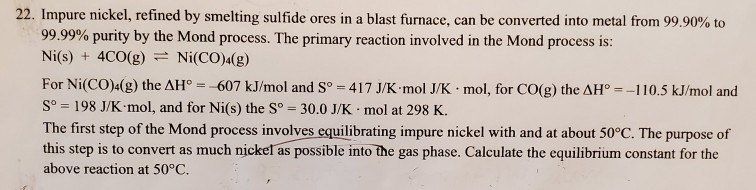22. Impure nickel, refined by smelting sulfide ores in a blast furnace, can be converted into metal from 99.90% to 99.99% purity by the Mond process. The primary reaction involved in the Mond process is: Ni(s) + 4CO(g) = Ni(CO)4(g) For Ni(CO) (g) the AH° = 607 kJ/mol and Sº - 417 J/K mol J/ Kmol, for CO(g) the AH = -110.5 kJ/mol and Sº = 198 J/K mol, and for Ni(s) the Sº = 30.0 J/K.mol at 298 K. The first step of the Mond process involves equilibrating impure nickel with and at about 50°C. The purpose of this step is to convert as much nickel as possible into the gas phase. Calculate the equilibrium constant for the above reaction at 50°C.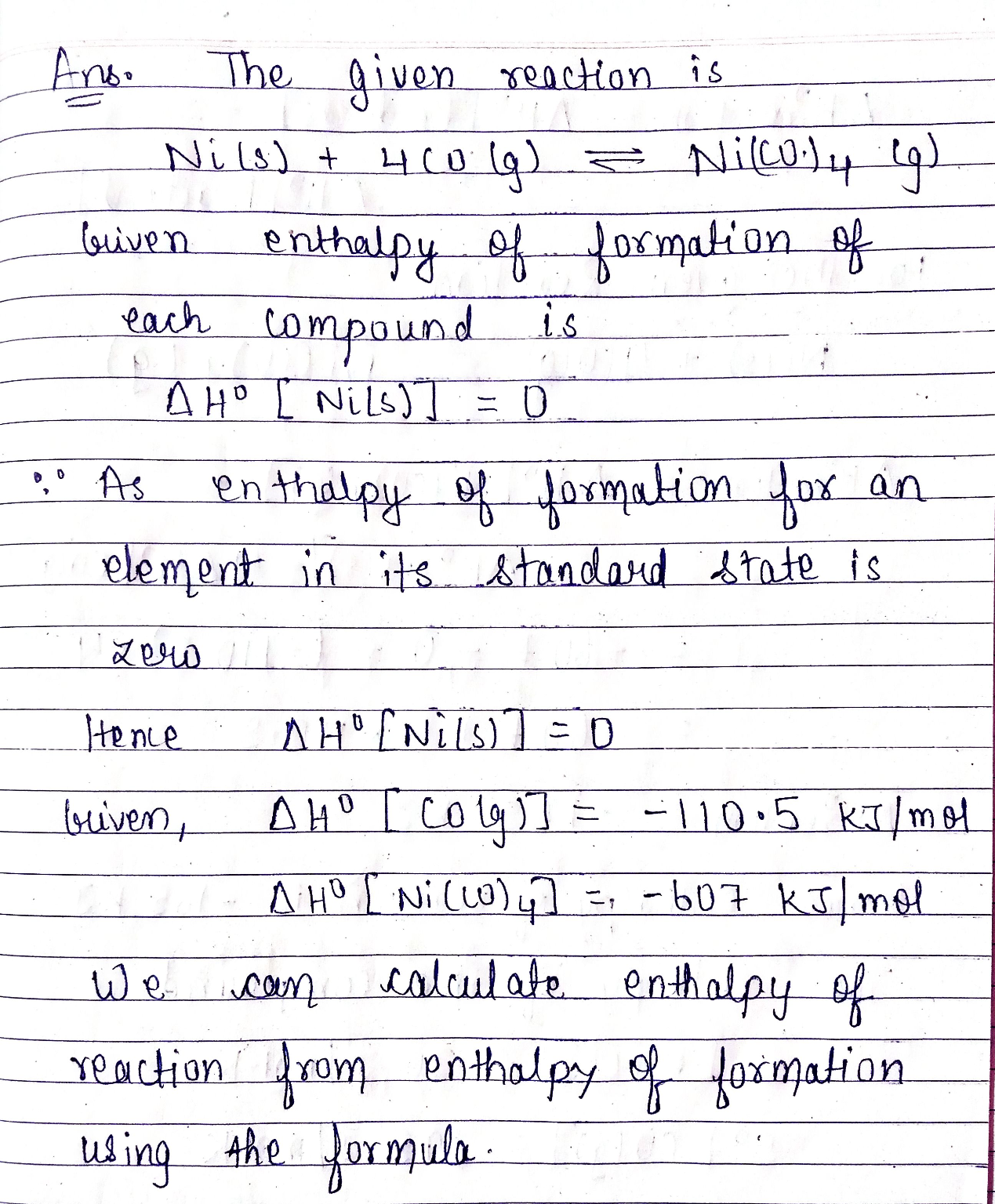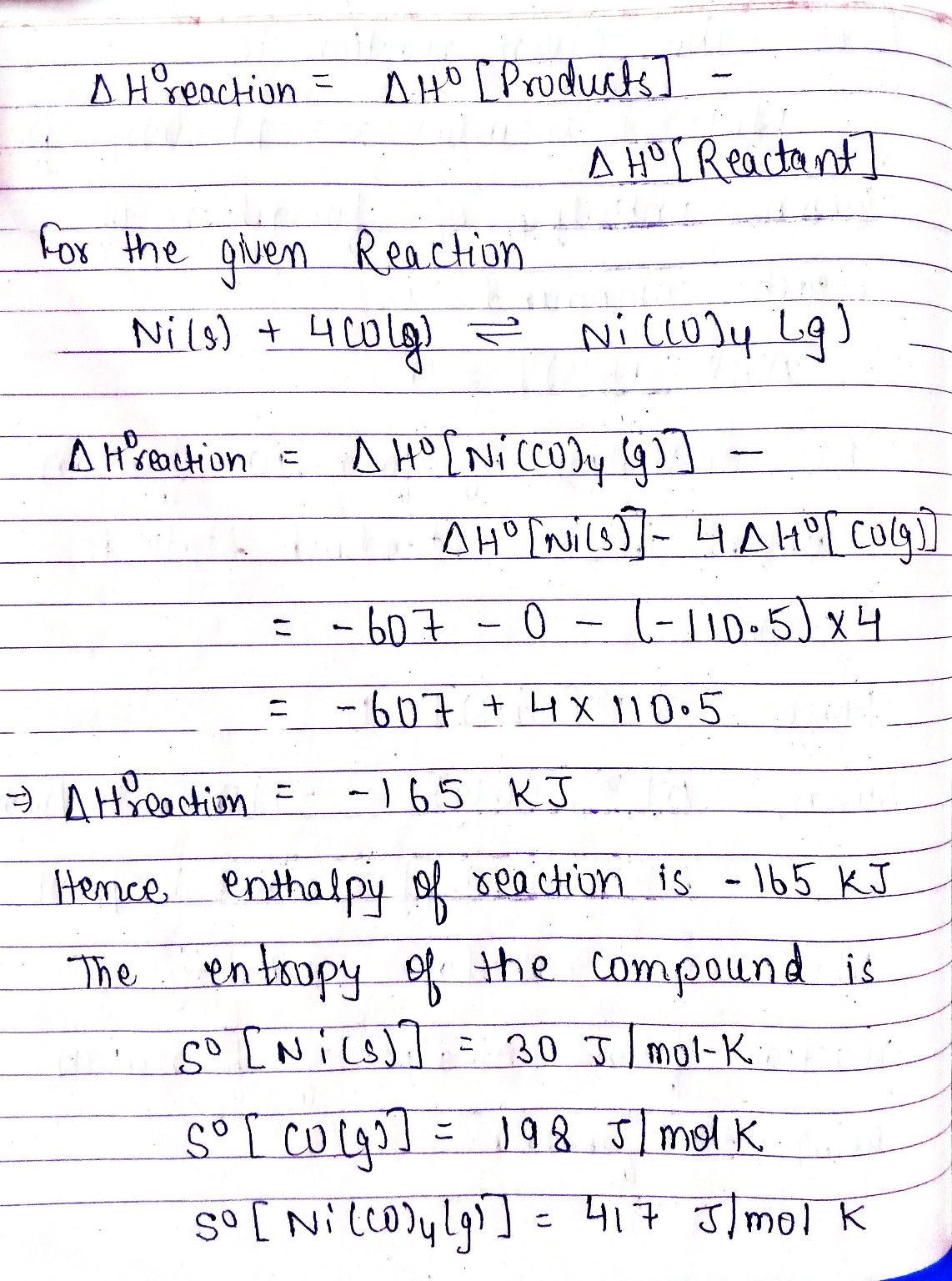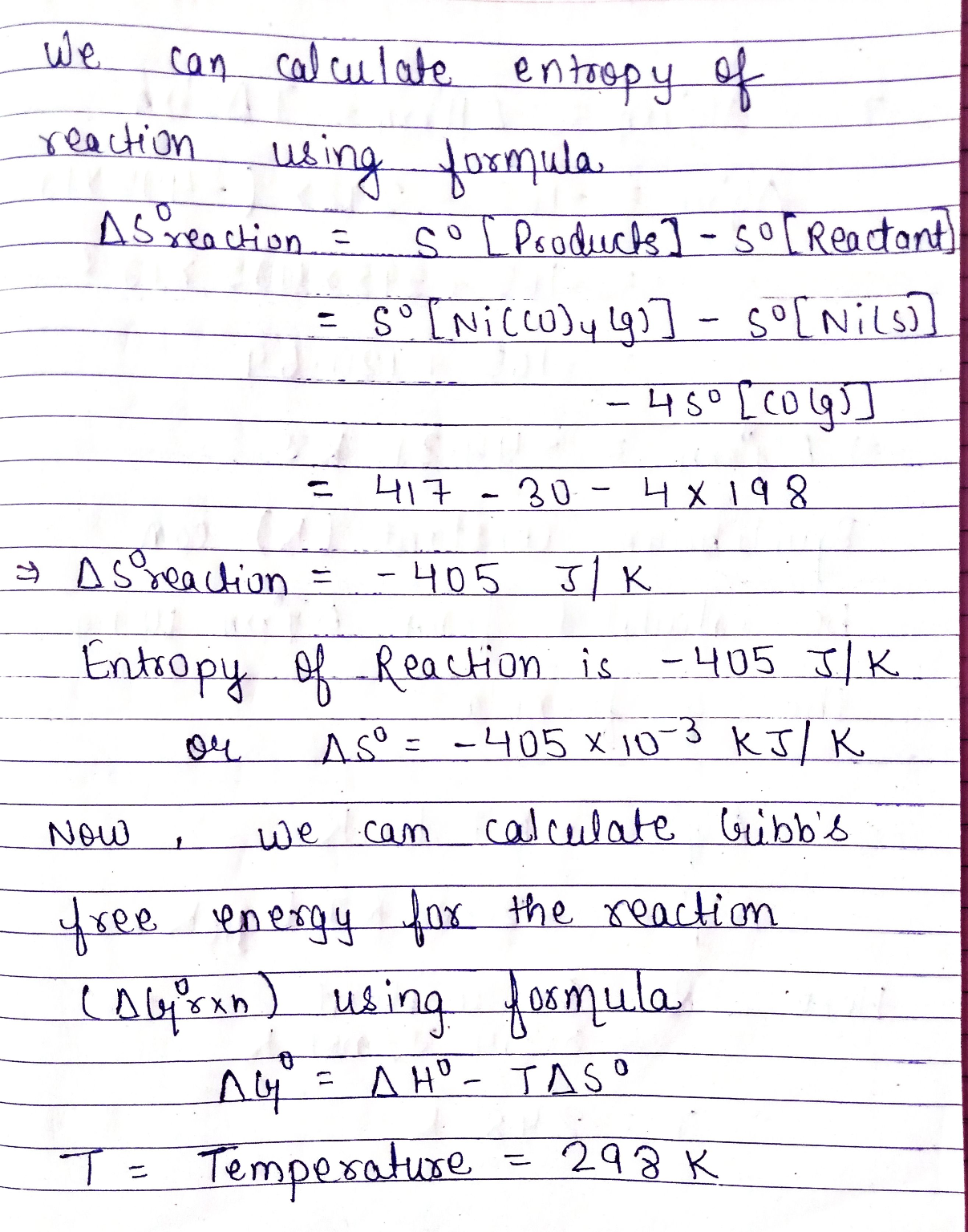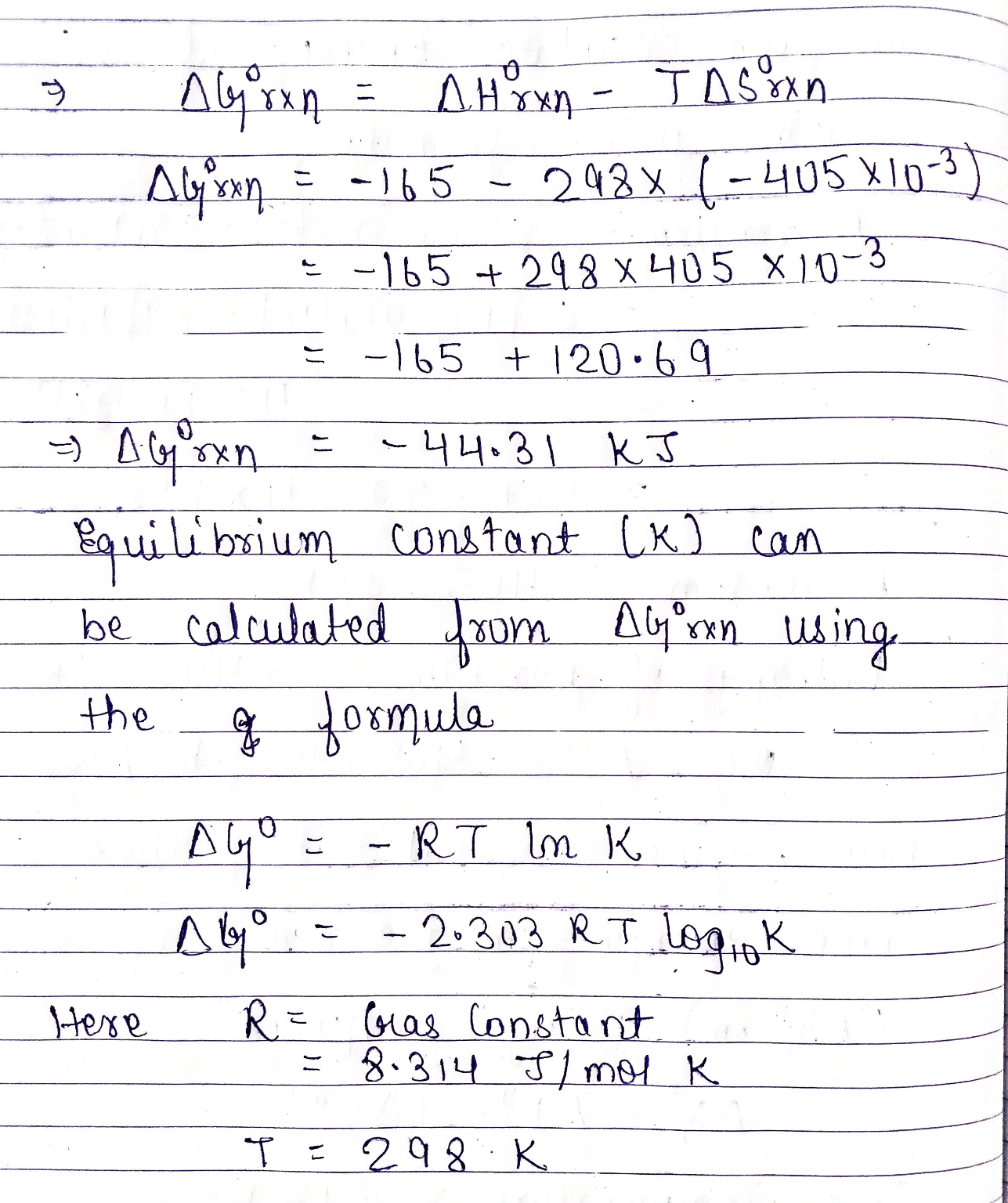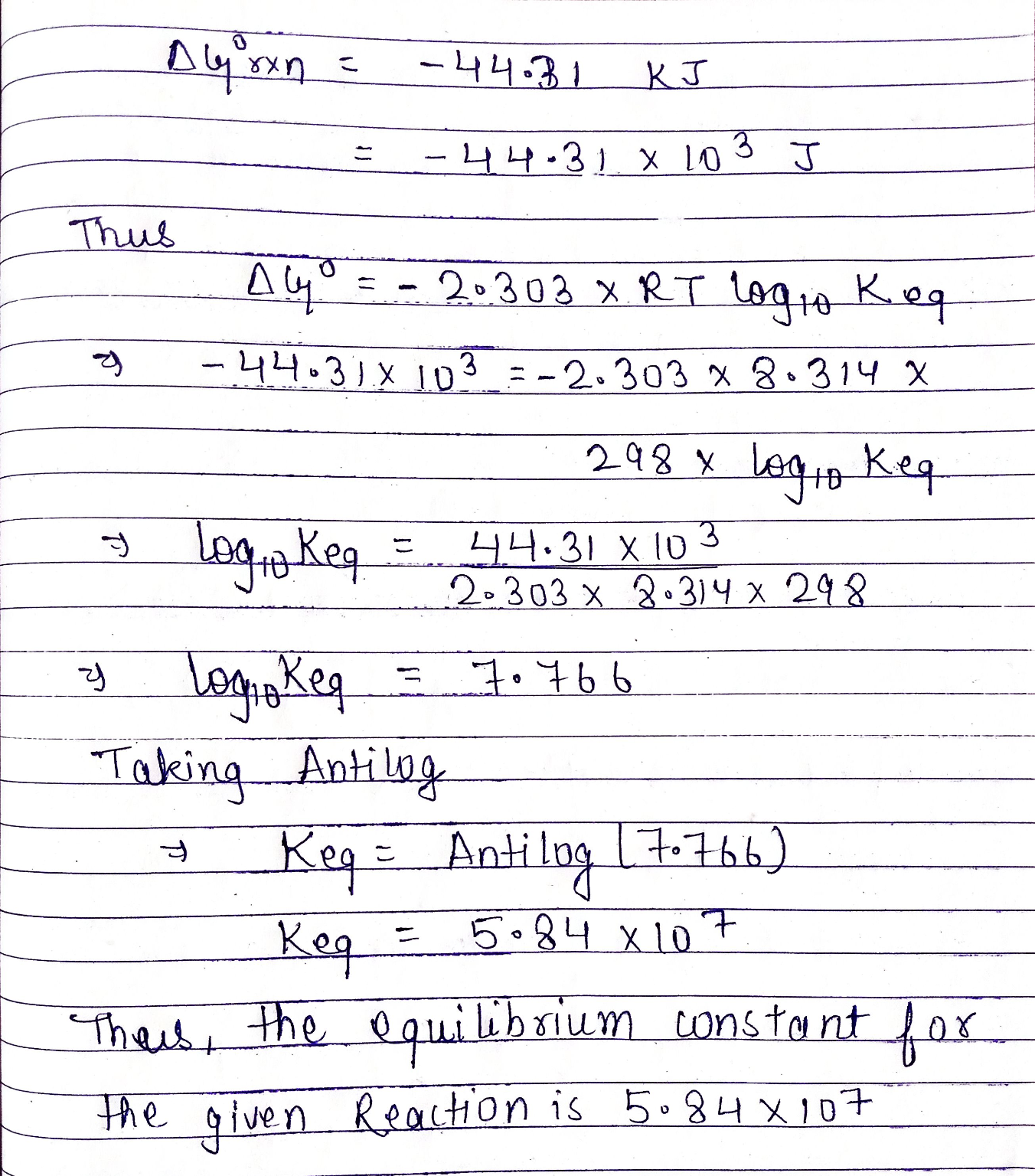Equilibrium constant , K = 5.84 ×107

#### Earn Coin

Coins can be redeemed for fabulous gifts.

Similar Homework Help Questions
• ### 3. Impure nickel, refined by smelting sulfide ores in a blast furnace, can be converted into...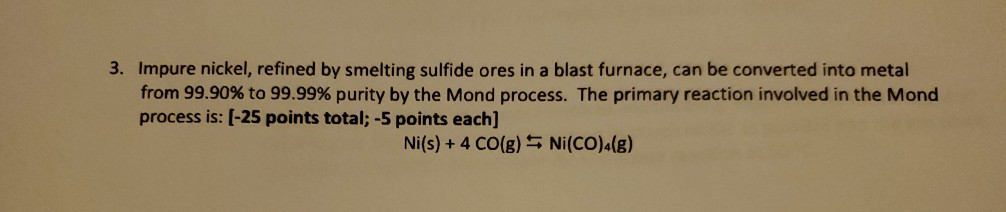3. Impure nickel, refined by smelting sulfide ores in a blast furnace, can be converted into metal from 99.90% to 99.99% purity by the Mond process. The primary reaction involved in the Mond process is: (-25 points total; -5 points each) Ni(s) + 4 CO(g) Ni(CO)4(8) a. The spontaneity of the above reaction is temperature dependent. Given the following values and those in the Appendix in your book, calculate Hº and ASº for the above reaction. [AH °(Ni(CO).) = -607...

• ### Purification of Ni metal can be facilitated by its ability to react with carbon monoxide. Ni(s)...

Purification of Ni metal can be facilitated by its ability to react with carbon monoxide. Ni(s) + 4CO(g) --> Ni(CO)4(g) Substance: Ni(s) CO(g) Ni(CO)4(g) S°(J/K•mol): 30.1 197.9 410.6 DH° (kJ/mol): 0 -110.5 -602.9 What is DG° for this reaction? A) –437.9 kJ/K•mol B) +437.9 kJ/K•mol C) +38.4 kJ/K•mol D) –38.4 kJ/K•mol E) –438.2 kJ/K•mol

• ### A. In the second step of the Mond process for the purification of nickel, gaseous Ni(CO)4...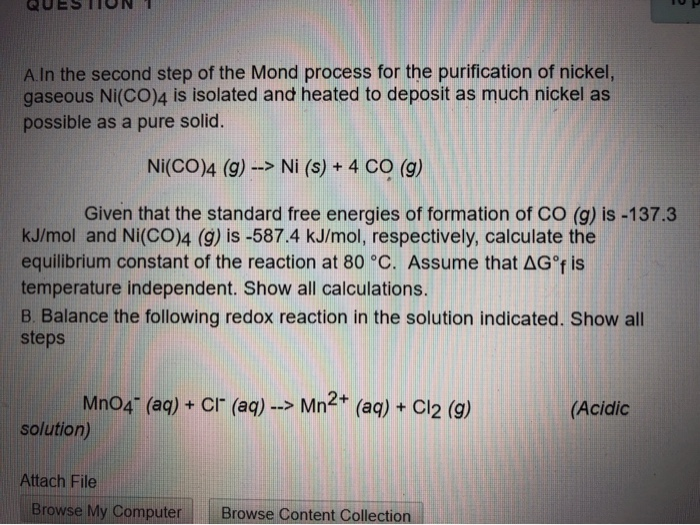A. In the second step of the Mond process for the purification of nickel, gaseous Ni(CO)4 is isolated and heated to deposit as much nickel as possible as a pure solid. Ni(CO)4 (9) --> Ni (s) + 4 CO (g) Given that the standard free energies of formation of CO (g) is -137.3 kJ/mol and Ni(CO)4 (9) is -587.4 kJ/mol, respectively, calculate the equilibrium constant of the reaction at 80 °C. Assume that AG°f is temperature independent. Show all calculations....

• ### Which steps in the above process can be represented by the following potential energy diagram? Debon...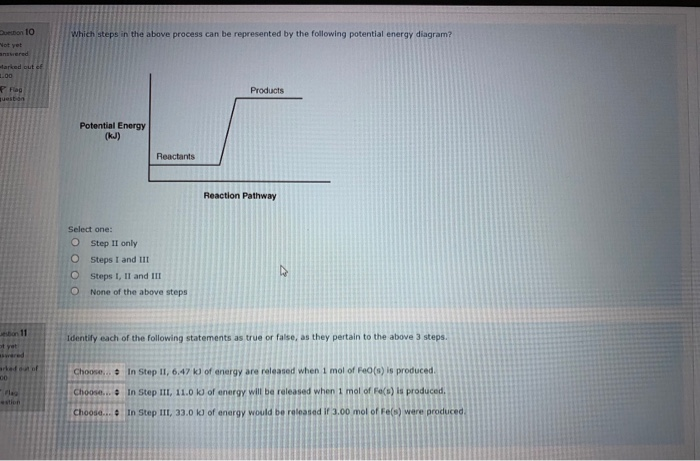Which steps in the above process can be represented by the following potential energy diagram? Debon 10 Not you wered Marked out of Products Potential Energy (kJ) Reactants Reaction Pathway Select one: Step II only O Steps I and II O Steps I, II and II None of the above steps Identify each of the following statements as true or false, as they pertain to the above 3 steps. Choose... In Step II, 6.47 kl of energy are released when...

• ### Use Table 6 values to calculate AGº (in kJ) at 2000 K for the following reaction:...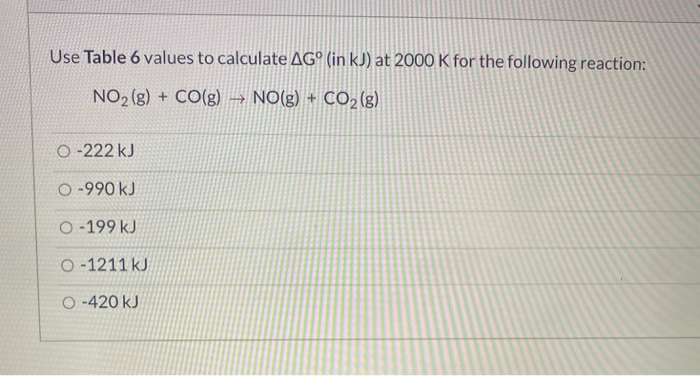Use Table 6 values to calculate AGº (in kJ) at 2000 K for the following reaction: NO2(g) + CO(g) NO(g) + CO2(g) 0 -222 kJ 0-990 kJ 0 - 199 kJ O-1211 kJ O-420 kJ AG; (kJ/mol ) at 25°c AH; Sº (kJ/mol) (KJ/K mol) AG, (kJ/mol) at 25°c ΔΗ: sº (kJ/mol) (kJ/kmol) 0.0 -20.6 H2S (g) -33.6 +0.2057 -814.0 H2SO4(0) +0.1569 -690.1 0.0 Hg(e) 0.0 +0.0760 -90.8 Hgo (3) -58.6 +0.0703 12 () 0.0 0.0 +0.1161 0.0 K(s) +0.0642...

• ### Please help with #17!!!! Example 17: Fill in the chart: 101 Version A Spring 2019 This...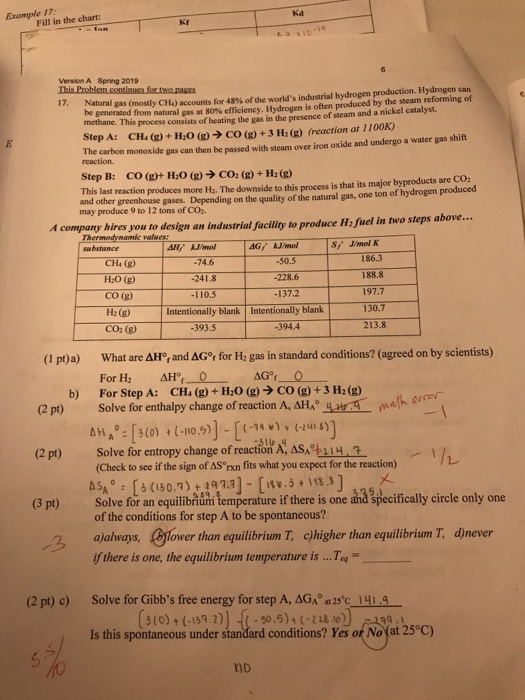Please help with #17!!!! Example 17: Fill in the chart: 101 Version A Spring 2019 This Problem continues for twe.pages Natural gas ( mostly CH.) accounts for 48% of the world's industrial hydrogen production. Hydrogen can be generated from natural as a 80efficiency. Hydrogen is often produced by the steam reforming of methane. This process consists of heating the gas in the presence of steam and a nickel catalyst Step A: CH. (g) + H2O(g) → CO(g) + 3 H2(g)...

• ### Use Table 6 values to calculate AGº (in kJ) at 2000 K for the following reaction:...Use Table 6 values to calculate AGº (in kJ) at 2000 K for the following reaction: NO2(g) + CO(g) + NO(g) + CO2(g) O-420 kJ 0-222 kJ O-1211 kJ 0-199 kJ 0-990 kJ 3 pts Question 27 What is the pH of .731 M H2CO3 (aq)? 8.4 O 5.2 O 3.3 O 6.5 10.7 Table 6 - Thermodynamic Data of 12.0 M HOH ZOH is added to Ag(s) AgBr(s) Agcl(s) AgI (8) AgNO, () Ag20(8) Al(a) Al,0, () Ba (3)...

• ### help with the following combustion reaction. shown are the tables and answer. I am confused with...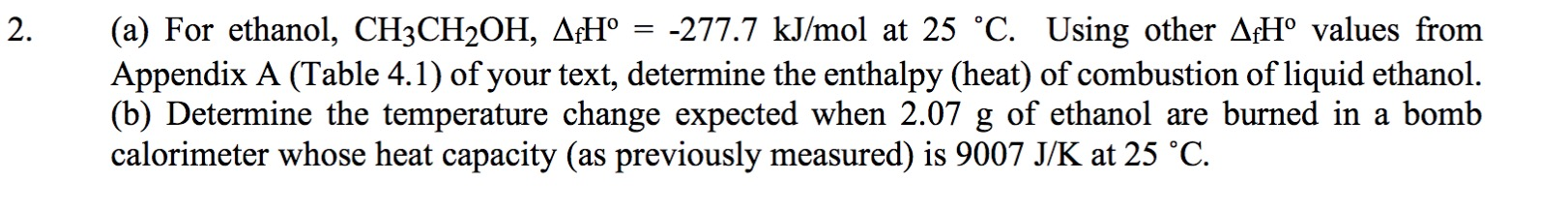help with the following combustion reaction. shown are the tables and answer. I am confused with the steps to get there. 2. (a) For ethanol, CH3CH2OH, AfHº = -277.7 kJ/mol at 25 °C. Using other AfHº values from Appendix A (Table 4.1) of your text, determine the enthalpy (heat) of combustion of liquid ethanol. (b) Determine the temperature change expected when 2.07 g of ethanol are burned in a bomb calorimeter whose heat capacity (as previously measured) is 9007 J/K...

• ### Using enthalpies of formation (Appendix C), calculate ΔH ° for the following reaction at 25°C. Also...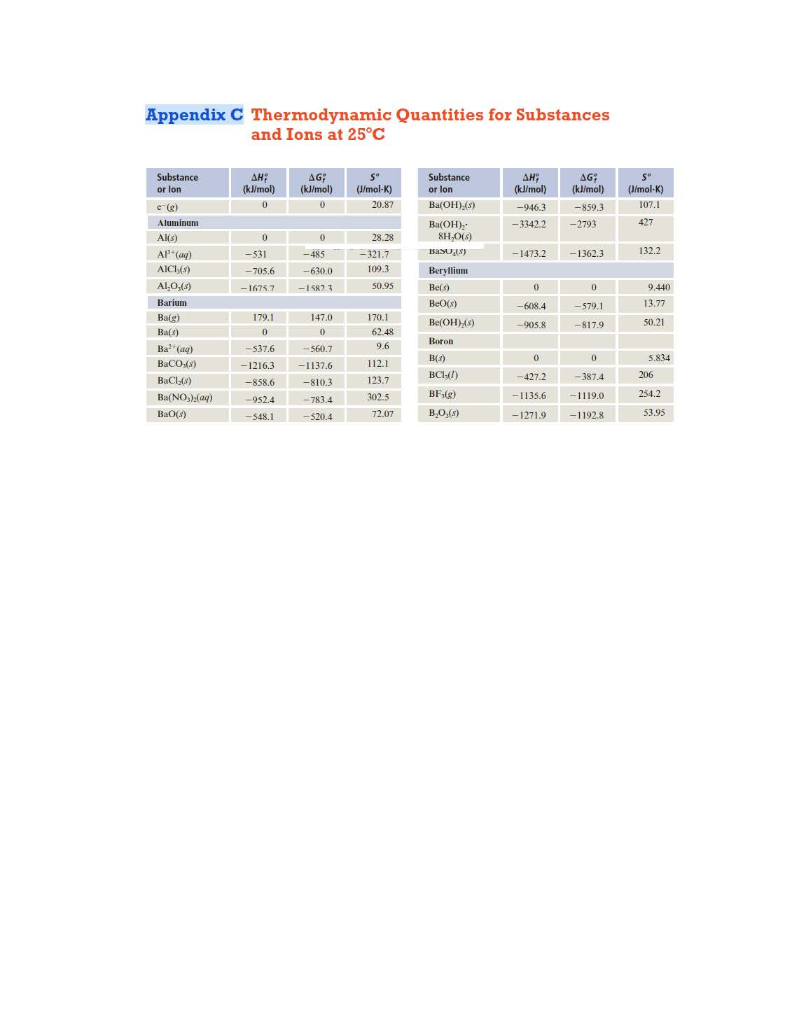Using enthalpies of formation (Appendix C), calculate ΔH ° for the following reaction at 25°C. Also calculate ΔS ° for this reaction from standard entropies at 25°C. Use these values to calculate ΔG ° for the reaction at this temperature. COCl2(g) + H2O(l ) h CO2(g) + 2HCl(g). Appendix C Thermodynamic Quantities for Substances and Ions at 25°C Substance or lon AH; (kW/mol) 0 AG: (kJ/mol) 0 S° (J/mol-K) 20.87 ΔΗ, (kJ/mol) -946.3 - 33422 AG; {kj/mol) --859,3 -2793 (J/mol-K)...

• ### 1. Calculate AHⓇ and AGº, using data in Appendix C. Determine whether each reaction is endothermic or exotherm...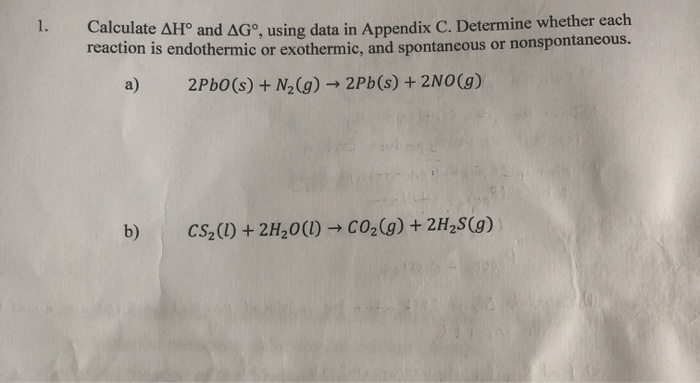1. Calculate AHⓇ and AGº, using data in Appendix C. Determine whether each reaction is endothermic or exothermic, and spontaneous or nonspontaneous. a) 2PbO(s) + N2(g) → 2Pb(s) + 2NO(g) b) CS2(1) + 2H2O(l) + CO2(g) + 2H2S(9) vendix C Thermodynamic Quantities for Substances and Ions at 25°C Substance or lon ΔΗ? (kJ/mol) sº AG; (kJ/mol 0 (J/mol-K) 20.87 ΔΗ: (kJ/mol) AG; (kJ/mol) -859.3 -2793 (J/mol-K) 107.1 Substance or lon Ba(OH),(s) Ba(OH), 8H 0(3) BaSO.() -946.3 -3342.2 427 0 -...